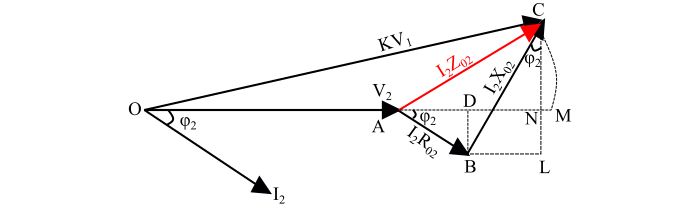# Approximate Voltage Drop in a Transformer and Voltage regulation of a Transformer

## Approximate Voltage Drop in a Transformer

The figure shows the approximate equivalent circuit of a practical transformer referred to secondary side.At no-load i.e. with I2= 0, the secondary terminal voltage is,

$$\mathrm{V_{2}=KV_{1} .....(1)}$$

When an inductive load of power factor cosφ2 (lagging) is connected across the secondary winding of the transformer, the secondary winding carries a current I2 and hence the voltage drops occur in $(R_{2} + K^2R_{1})$ and $(X_{2} + K^2X_{1})$. As a result, the secondary voltage drops from KV1 to V2 across the load terminals.

By applying KVL to the circuit, we get,

$$\mathrm{V_{2}=KV_{1}-I_{2}[(R_{2} + K^2R_{1})+J(X_{2} + K^2X_{1})]}$$

$$\mathrm{⇒ V_{2}=KV_{1}-I_{2}(R_{02} +jX_{02}) = KV_{1}-I_{2}Z_{02}}$$

Therefore, the drop in secondary voltage is,

$$\mathrm{ KV_{1}-V_{2}=I_{2}Z_{02} .....(2)}$$Now, referring the phasor diagram, it is clear that the drop in the secondary voltage is $AC = I_{2}Z_{02}$, which can be determined as follows

With O as centre and OC as radius, an arc OA is cutting and produced at M, then,

$$\mathrm{AC=AM \approx AN}$$

Now, from B, draw BD which is perpendicular to OA, draw CN which is perpendicular to OM and draw BL which is parallel to OM. Therefore, the approximate drop in the secondary voltage is given by,

$$\mathrm{Approximate\:drop\:in\:secondary\:voltage= AN = AD+DN}$$

$$\mathrm{\therefore \:BL = DN}$$

$$\mathrm{\because \:AN = AD + BL}$$

$$\mathrm{⇒ AN = I_{2}R_{02}\:cos\:\varphi _{2}\: + I_{2}X_{02}\:sin\:\varphi _{2} ....(3)}$$

The eqn. (3) gives the approximate drop in secondary voltage for the load of lagging power factor.

For a load of leading power factor, the approximate voltage drop of the transformer is given by,

$$\mathrm{Approximate\:voltage\:drop\:=I_{2}R_{02}\:cos\:\varphi _{2}\:-\:I_{2}X_{02}\:sin\:\varphi _{2} ....(4)}$$>

Also, if the circuit of the transformer being referred to primary side, then

$$\mathrm{Approximate\:voltage\:drop\:= I_{1}R_{01}\:cos\:\varphi_{1}\:±\:I_{1}X_{01}\:sin\:\varphi _{1} ....(5) }$$

Since, the difference between the value of primary power factor (cos φ1) and the secondary power (cos φ2) is very small. Therefore, the eqn. (5) can also be written as

$$\mathrm{Approximate\:voltage\:drop\:=\:I_{1}R_{01}\:cos\:\varphi _{2}\: ±\:I_{1}X_{01}\:sin\:\varphi _{2} ....(6)}$$

Here, the positive sign is used for a load having lagging power and the negative sign for the load of leading power factor.

## Voltage Regulation of Transformer

The voltage regulation of a transformer is defined as the arithmetic difference between the no-load secondary terminal voltage (V2NL) and full-load secondary terminal voltage (V2FL) expressed as a percentage of full-load (rated) secondary terminal voltage at a given power factor with a constant value of primary voltage for both no-load and full-load i.e.

$$\mathrm{Voltage\:regulation\:= \frac{V_{2NL}\:-\:V_{2FL}}{V_{2FL}}\:*100 ..... (7) }$$

From the eqn. (2), the no-load secondary terminal voltage is

$$V_{2NL} = KV_{1}$$

Therefore, the voltage drop in the transformer from no-load to full load is given by,

$$\mathrm{V_{2NL}\:-\:V_{2FL}\:=\: I_{2}R_{02}\:cos\:\varphi _{2}\:±\:I_{2}X_{02}\:sin\:\varphi _{2} .....(8)}$$

Where, the positive sign is used for lagging power factor load whereas the negative sign for load having leading power factor.

$$\mathrm{\therefore\:Voltage\:regulation\:=\:\frac{I_{2}R_{02}\:cos\:\varphi_{2}\:±\:I_{2}X_{02}\:sin\:\varphi _{2}}{V_{2FL}} ......(9)}$$

The voltage regulation is the measure of performance of the transformer. For an ideal transformer the voltage regulation is zero. For the better operation of the transformer, the voltage regulation should be as small as possible.

For a transformer the limits of variation in the voltage being specified in terms of voltage regulation. For example, the variation in the terminal voltage of the transformer used in public supply system must not exceed ± 5%.

## Condition for Zero Voltage Regulation

Consider an inductive load is connected across the transformer, then the voltage regulation of the transformer is

$$\mathrm{\therefore\:Voltage\:regulation\:=\:\frac{I_{2}R_{02}\:cos\:\varphi _{2}\:+\:I_{2}X_{02}\:sin\:\varphi _{2}}{V_{2FL}} ..... (9)}$$

From this expression, it can be seen that the voltage regulation will be zero when the numerator is zero i.e.

$$\mathrm{I_{2}R_{02}\:cos\:\varphi _{2}\:+\:I_{2}X_{02}\:sin\:\varphi_{2}= 0}$$

$$\mathrm{⇒\:tan\:\varphi_{2}\:=\:-\frac{R_{02}}{X_{02}}}$$

$$\mathrm{⇒\:\varphi_{2}\:=\:-tan^-1(\frac{R_{02}}{X_{02}}) .....(10)}$$

Here, the negative indicates that zero voltage regulation occurs when the load is capacitive, i.e., having leading power factor.